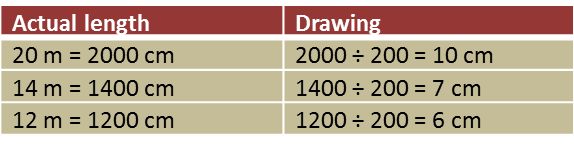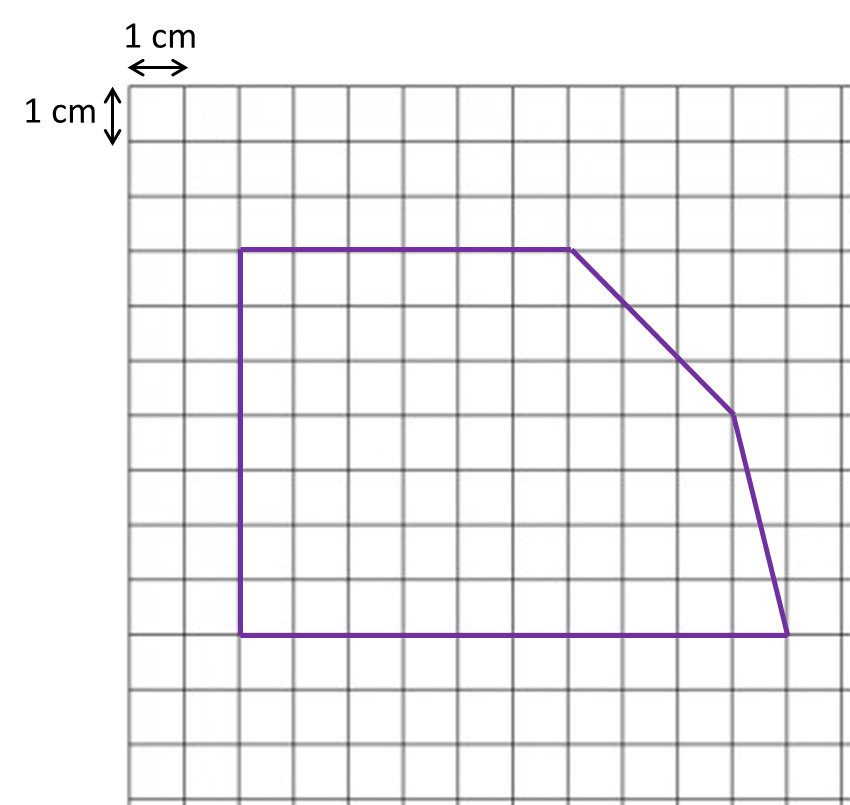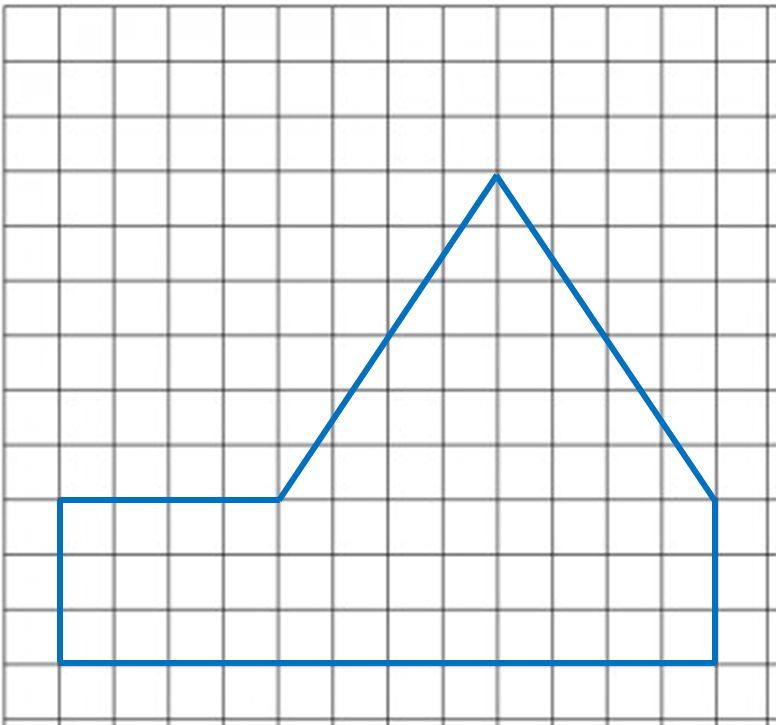9.2.2 Scale Drawings, PT3 Focus Practice

Question 6:
Diagram below shows an irregular pentagon.By using the scale 1 : 200, complete the scale drawing in the answer space provided. The grid has equal square with sides of 1 cm.Solution:Question 7:
Diagram below shows polygon.(a) If the polygon is redrawn using the scale 1 : 500, calculate the length of side drawn for the side 15 m.
(b) On the square grids in the answer space, redraw the polygon using the scale 1 : 500. The grid has equal squares with sides of 1 cm.